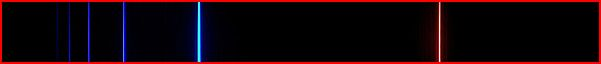Home | Study Links | Rhymes |

## Balmer and RydbergIdeas and adjusted constants for the Balmer formula and Rydberg equations.

While investigating time lines and dates using certain numbers from the book of Daniel as mentioned in the Bible, it occurred to me that the number 364.5 (a number involved in other calculations) also happens to be the Balmer constant number 364.5, used in measuring spectral rays and 'time lines' in the light and electromagnetic spectrum.

Balmer had noticed that a single number had a relation to every line in the hydrogen spectrum that was in the visible light region, and that number was "364.56" nm.
"The Balmer series or Balmer lines in atomic physics, is the designation of one of a set of six different named series describing the spectral lines emission of the hydrogen atom. The Balmer series is calculated using the Balmer formula, an empirical equation discovered by Johann Balmer in 1885. "Wikipedia"

When the "1260" Daniels days are divided by 364.5, the answer became: 3.4567_901234567_901234567_9...etc.
(All numbers 8 are missing)! However, when 364.500117160... is used the number string becomes "complete" when the number eight is included: 3.4567*8*901234567*8*901234567*8*901234567*8*9...

So by dividing this "complete" number string into 1260, you may end up with an accurate Balmer constant number! It seems to be saying that 364.5 is very close, but here is confirmation that 364.500117... is exact!

The Balmer constant "364.56" and Rydberg constant "1.097393690", are the approximation numbers used today.
Balmer constant:    364.56"
Rydberg constant:  1.097393690"

364.50 x 4 / 1.2= 1215
364.56 x 4 / 1.2= 1215.2
364.500117160... x 4 / 1.2= 1215.00039053.../ 1000 x 300= 364.500117160...

1.2 mirrors 2.1 and 2.1 / 1.2 = 1.75 x 4 = 7
1.2 is also the result of the division between top and bottom half measurements of the "diamond" shape.

4 / 364.5 x 100= 1.097393690 (Rydberg constant)
(1.3333 / 1.215000390536... = 1.09739333725...) or (400 / 364.500117160...)

364.500117160… x 1.0973933372525... = 400 (Exact)
364.56 x 1.097393690 = 400.0658436... (Not quite)
364.50 / 4 = 91.125
364.56 / 4 = 91.14
364.500117160…/ 4 = 91.125029290… continue to divide by 2 until 2.8476571653... X 1.09739333… = 3.125
3125 units at 3.2 π, is the height to the Pyramid tip representing the limit of the physical realm!
125 / 39.062500 = 3.2
125 / 40              = 3.125
125 / 39.78860...= "π 3.1416026…"
364.5 x 2 (9X) = 4322 or c light speed (186624 mps).

Wavelengths: Balmer Constant adjusted to 364.500117160…
Visible range:
(364.500… x 32) / (4 - 32) = 656.1002 or (364.5001… x 3.6 / 2 or (1.8) Color: Red
(364.500… x 42) / (4 – 42) = 486.0001 (divided by 1.333333333 = 364.5001…) Color Blue/green
(364.500… x 52) / (4 – 52) = 433.9287 Violet
(364.500… x 62) / (4 – 62) = 410.0626 Violet
Ultraviolet range:
72 = 396.9001…
82 = 388.8001…
92 = 383.4351…
102 = 379.6896…
112 = 376.9616…
122 = 374.9144…
132 = 373.3364…
142 = 372.0938…
152 = 371.0974…
162 = 370.2858…
172 = 369.6159…
182 = 369.0563…
192 = 368.5841…
202 = 368.1819…
364.500… x 212 = 404.8981150… cancel out # 4 and answer is 364.500117160… Balmer's constant.
*4* - 212
222 = 364.500…
232 = 364.500…
Etc.

Small sections of the Electromagnetic spectrum based on numbers viewed in a mathematical and geometric way. The entire spectrum from the center and continuing in opposite directions is divided into sections of 37 whether .37 or 37 etc., (macro and micro), apparently in an extremely organized way. For example 1.11 has 3 sections of .37, 111= 300, 222= 600 etc.

Multipling numbers by the new Rydberg constant number 1.0973933372525... :
.999= 1.096295943915343…
1.00= 1.097393337252596…
1.01= 1.108367270625122…
1.02= 1.119341203997648…
1.03= 1.130315137370174…
1.04= 1.141289070742700…
1.05= 1.152263004115226… >>etc.
1.06= 1.163236937487752…
1.07= 1.174210870860278…
1.08= 1.185184804232804…
1.09= 1.196158737605330…
1.10= 1.207132670977856…
1.11= 1.218106604350382…
1.12= 1.229080537722908…

Perhaps this expanding and spiraling sheet of numbers in the electromagnetic spectrum contains interesting patterns, and made more discernable if the numbers (0-9) had their own colors.

The numbers 1 to 9 add to 45, and the numbers 1 to 9 minus 8 add to 37. So 45 / 37 = 1.216216216..., led to my thinking about fine-tuning possibilities for the Rydberg equation and the hydrogen's number of 1.097393690...